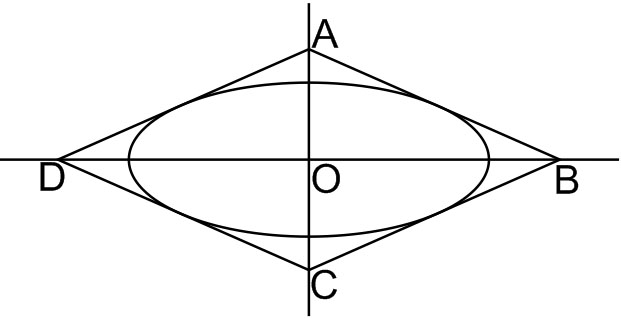#### The area (in sq. units) of the quadrilateral formed by the tangents at the end points of the latera recta to the ellipseOption 1)Option 2) 18 Option 3)Option 4) 27As we have discussed in

Length of latus rectum of ellipse -- wherein

For the ellipseCoordinates of foci -- wherein

For the ellipseEccentricity -- wherein

For the ellipseEquation of tangent isa2 = 9, b2 = 5Hence, endpoints of LRs =Thus, equation of one of tangent isIf y = 0If x = 0Hence, area ofArea of quadOption 1)This option is incorrect.

Option 2)

18

This option is incorrect.

Option 3)This option is incorrect.

Option 4)

27

This option is correct.

#### perimeter